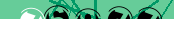# JavaScript: Objects with constructors

With the advent of ES6 there are a confusing number of ways to create and invoke objects/classes in JavaScript. Here we're presenting four short examples of the same simple object/class using different notation.

## Object as constructor function

Perhaps the simplest to make sense of is the class-as-function model. One advantage is that variables defined with var will be kept private and inaccessible from outside the class:

```// define Pizza function Pizza(slices) { // private variable var slices = parseInt(slices); // private method var hasSlices = function() { return slices > 0; } // public method this.takeSlice = function() { if(hasSlices()) { slices--; console.log("you took a slice"); return true; } console.log("no pizza for you!"); return false; } } // create an instance of Pizza var pizza = new Pizza(8); // take slices until they run out do { console.log("I'm hungry"); } while(pizza.takeSlice());```

The do .. while segment is common to all examples on this page. The output is identical in each case and should be predicatable, or you can run the code yourself to find out.

In this model if we examine the pizza variable we only see:

`Pizza {takeSlice: function}`

Any outside references to pizza.slices or pizza.hasSlices() will return "undefined". Variables don't have to be private, in which case you use this.slices throughout, as in the examples below.

The drawback with this approach is that every instance of Pizza comes with a copy of all its methods, and if you want to retroactively add or update a method it has to be done again for every instance.

## Literal notation

Using literal (:) notation we can only ever have a single instance of our class. There is no constructor as such, so we need an init() method to serve that purpose:

```// define pizza as a variable var pizza = { init: function(slices) { this.slices = parseInt(slices); }, hasSlices: function() { return this.slices > 0; }, takeSlice: function() { if(this.hasSlices()) { this.slices--; console.log("you took a slice"); return true; } console.log("no pizza for you!"); return false; }, } // initialize pizza pizza.init(8); do { console.log("I'm hungry"); } while(pizza.takeSlice());```

Examining the pizza object in this case returns all attributes and methods (nothing is private):

`{init: function, hasSlices: function, takeSlice: function, slices: 0}`

This means that from outside the class we can do something crazy like:

`pizza.slices = 1000;`

## Hybrid notation

This one is a bit strange, an object constructor with private variables and methods, returning an oject with public methods for accessing those private properties:

```// define Pizza var Pizza = function(slices) { // private variable var slices = parseInt(slices); // private method var hasSlices = function() { return slices > 0; }; return { // public method takeSlice: function() { if(hasSlices()) { slices--; console.log("you took a slice"); return true; } console.log("no pizza for you!"); return false; } }; } // initialize pizza var pizza = new Pizza(8); do { console.log("I'm hungry"); } while(pizza.takeSlice());```

When examining our object we can only see the public method:

`{takeSlice: function}`

This difference between this and our first example is that pizza is no longer an instance of Pizza. Instead it is just an anonymouse object returned by the Pizza constructor.

## ES5 Prototyping

In JavaScript ES5 classes are created using prototyping. You stare with a base Object and start adding methods:

```// define Pizza function Pizza(slices) { this.slices = parseInt(slices); } Pizza.prototype.hasSlices = function() { return this.slices > 0; } Pizza.prototype.takeSlice = function() { if(this.hasSlices()) { this.slices--; console.log("you took a slice"); return true; } console.log("no pizza for you!"); return false; }; // create an instance of Pizza var pizza = new Pizza(8); do { console.log("I'm hungry"); } while(pizza.takeSlice());```

Again, we can see that all attributes of the class are public:

`Pizza {slices: 8, hasSlices: function, takeSlice: function}`

There is no (simple) way to define a private variable as we did earlier.

Prototyping is useful in that when you modify the behaviour of a class all previously created instances will have access to the new or updated methods.

There are also memory savings as the prototyped methods are only loaded once even if you instantiate hundreds of objects of the same type.

## ES6 Class Notation

JavaScript ES6 gives us a new syntax where the class definition is wrapped in a single stanza and can have a constructor. The syntax is deceptively close to PHP:

```// define Pizza class class Pizza { constructor(slices) { this.slices = parseInt(slices); } hasSlices() { return this.slices > 0; } takeSlice() { if(this.slices >= 1) { this.slices--; console.log("you took a slice"); return true; } console.log("no pizza for you!"); return false; } } // create an instance of Pizza var pizza = new Pizza(8); do { console.log("I'm hungry"); } while(pizza.takeSlice());```

But there's still no concept of private variables:

```Pizza { slices: 8 } Pizza Prototype { constructor: function() hasSlices() takeSlice() }```

Only in the next version, currently referred to as ESnext, will private variables be introduced, with variable names prefixed with the hash "#" symbol.

Otherwise the progression from functions to classes is mostly about improving performance, and enabling OOP programming techniques such as inheritance, but that's another story.

## ESnext proposed syntax

Using the new syntax we replace this.slices with this.#slices and the variable becomes private.

```// define Pizza class class Pizza { #slices; // declare private variable constructor(slices) { this.#slices = parseInt(slices); } // private method (draft proposal) #hasSlices() { return this.#slices > 0; } takeSlice() { if(this.#hasSlices()) { this.#slices--; console.log("you took a slice"); return true; } console.log("no pizza for you!"); return false; } }```

At the time of writing no browser supports this feature and it will generate a syntax error.

## Other ways to be private

If keeping variables private is a concern you can always encapsulate your code in:

```(function() { ... })();```

or:

```window.addEvenListener("DOMContentLoaded", function(e) { ... }, false);```

There are also any number of complicated schemes being discussed on stackoverflow.

## References

< JavaScript

#### Send a message to The Art of Web: